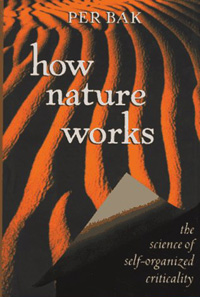# What Does BTW Stand for?

BTW =’by the way’ for the texting generation, but you did not come here for something so obvious, right?

Older physicists and mathematicians use the acronym to refer to a pair of fascinating papers from 1987 (here and here). They were written by Danish physicist Per Bak, and his colleagues Chao Tang and Kurt Wiesenfeld.

They were definitely among the most important papers I read in my life, because they showed how complex behavior can be obtained from simple mathematical models. Per Bak later wrote a popular physics book to explain the significance of his discovery.The best way to understand the model is to close all papers and books, and try the toy model yourself. Forget everything about sand, etc. You are essentially simulating a mathematical equation in cellular automata (i.e. digital) form.Even for those with mathematical background, it may take weeks to grasp the significance of the discovery. If you understand it too quickly, maybe you do not get it.

Why do we bring it up here? Biologists are trying to describe nature, but ‘describing nature’ essentially means fitting observations into known mathematical models. We hope increasing the toolbox of mathematical models will enhance the ability of researchers to describe many observations not fitting linear models.

Speaking of simple mathematical models, another book that blew our mind was Stephen Wolfram’s ‘A New Kind of Science’ (NKoS). The book got criticized, because Wolfram compared his book to be as significant as Newton’s Principia Mathematica. We thought his claims were accurate, but they seemed to have threatened many mediocre scientists.

Recent comments from Wolfram on NKoS -

[Living a Paradigm Shift: Looking Back on Reactions to A New Kind of Science

Its Been 10 Years: Whats Happened with A New Kind of Science?

Looking to the Future of A New Kind of Science

One interesting aspect of BTW and NKoS is that the models can be easily taught to young kids, even though the implications of the models go far deeper. A child born today will turn 30 in year 2042, but the mathematics we teach them is from 1842, if not before. How will they appreciate complexity of nature, if they are not introduced to the concepts at an early age?

Written by M. //